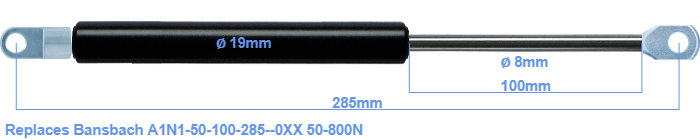# Replacement for Bansbach A1N1-50-100-285–0XX 50-800N

£28.23 (excl. VAT)

Replacement gas spring for the Bansbach A1N1-50-100-285--0XX 50-800 Newton. Mounting part tube: The eye has a thickness of 5mm and a hole diameter of 8.1mm. Mounting part rod : The eye has a thickness of 10mm and a hole diameter of 8.1mm. Brand: Hahn.

Please note:
• Mounting part tube: The thickness of the eye of this replacement is 5mm instead of 10mm.
• The diameter of the tube of this replacement gas spring is 19mm instead of 18mm.
•  Force Choose an option50 Newton60 Newton80 Newton100 Newton120 Newton140 Newton150 Newton160 Newton180 Newton200 Newton220 Newton240 Newton250 Newton260 Newton280 Newton300 Newton320 Newton340 Newton350 Newton360 Newton380 Newton400 Newton420 Newton440 Newton450 Newton460 Newton480 Newton500 Newton520 Newton540 Newton550 Newton560 Newton580 Newton600 Newton620 Newton640 Newton650 Newton660 Newton680 Newton700 Newton720 Newton740 Newton750 Newton760 Newton780 Newton800 NewtonClear
This gas spring is also known as Winco Easylift A1N1-50-100-285, A1N1 F50-100-285.